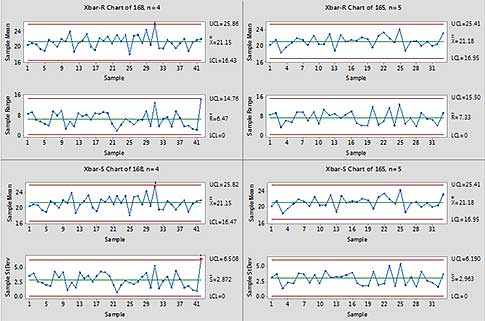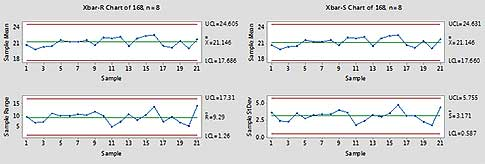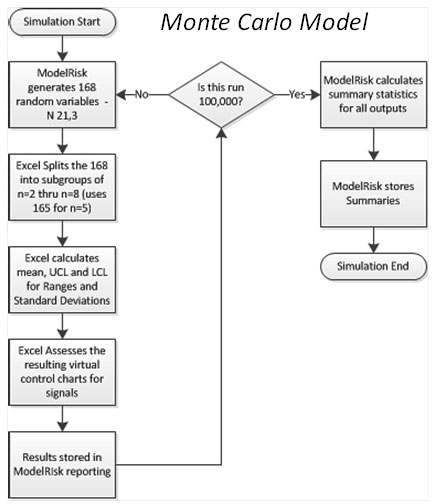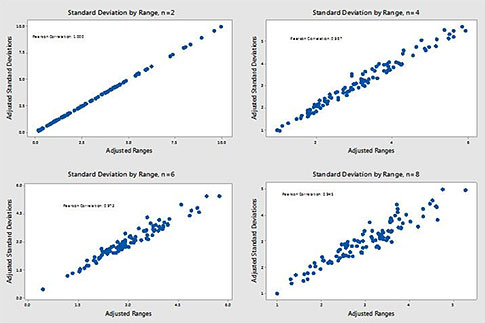#Quality Digest

Featured Product
This Week in Quality Digest Live
Six Sigma Features
Gregg Profozich
Six Sigma principles and tools
Gregg Profozich
Small and medium-sized manufacturers can improve their safety, quality, delivery, and costs with these methodologies
Jay Arthur—The KnowWare Man
Here’s a simple way to use Excel PivotTables to dig into your data
Anthony D. Burns
Upcoming interactive mobile app demonstrates Deming’s process variation experiment
Tom Taormina
Champion business success and avoid risk
Six Sigma News
Too often process enhancements occur in silos where there is little positive impact on the big picture
Collect measurements, visual defect information, simple Go/No-Go situations from any online device
Good quality is adding an average of 11 percent to organizations’ revenue growth
Floor symbols and decals create a SMART floor environment, adding visual organization to any environment
A guide for practitioners and managers
Making lean Six Sigma easier and adaptable to current workplaces
Gain visibility into real-time quality data to improve manufacturing process efficiency, quality, and profits
Makes it faster and easier to find and return tools to their proper places
Version 3.1 increases flexibility and ease of use with expanded data formatting featuresBio

Six Sigma

## Ranges vs. Standard Deviations: Which Way Should You Go?

### A look at the mathematical difference between X-bar R and X-bar S

Published: Tuesday, June 2, 2015 - 13:04

Recently, in one of the many online discussion groups about quality, Six Sigma, and lean, this question was posed: “Can X-bar R and X-bar S be used interchangeably based on samples size (n) if the subgroup size is greater than one and less than eight?” Answers varied, of course.

In some of these discussion groups, you get to see how far rule four of W. Edwards Deming’s funnel experiment has pushed some training programs off in one direction or another, especially when it comes to statistical process control (SPC). One set of answers that surprised me, though, came from a couple of consultants in France, who said, “Clearly not... the question is about a sample of 1 to 8. [The] response is definitely no. You can’t calculate a standard deviation with a sample of one or two. A sample higher than 8 is highly recommended.”

The point they were trying to make was that for subgroups of size eight  or smaller, you could only use X-bar R charts.

This was the first time I’d heard that claim. I have some statistician friends who will tell their students, “Since you have software, you might as well use the standard deviation, since it uses all the data.” That’s not my stance. I generally use the X-bar R chart until the sample size gets to 10, just because it’s an easier chart to teach, and an easier chart to use if you don’t have good SPC software. I had never heard anyone state that you must use an X-bar R for subgroup sizes smaller than nine, so I decided to play with it, just to see what the practical difference is between the charts (the mathematical difference).

I used Minitab to create a column of 168 random numbers from a normal distribution with a nominal mean of 21 and a nominal standard deviation of three. I then cut that column of data into subgroups of sizes two through eight (I only used the first 165 for subgroups if size five).

For subgroups of size two and three, the charts came out as in figure 1:Figure 1: X-bar R and X-bar S charts for subgroups of sizes two and three. Click here for larger image.

When n = 2, mean was 21.15, mean R-bar was 3.47, control limits for means were 14.62 and 27.67, and the UCL R-bar was 14.62. There was one subgroup mean outside the limits on the high side at subgroup 61, and one range outside at subgroup 83. For the X-bar S, mean S-bar was 2.54, control limits for means were 14.62 and 27.67, and the UCL S-bar was 8.015. There were the same signals at subgroups 61 and 83.

When n = 3, the mean R-bar was 5.38, control limits for means were 15.64 and 26.65, and the UCL R-bar was 13.85. One outside the control limits, this time at subgroup 41 (around the same high individuals as in the n = 2 chart); no out-of-control signals in the range chart. For X-bar S, the mean S-bar was 2.827, and control limits for means were 15.62 and 26.67 (0.02 tighter than limits based on ranges). The averages chart contained the same signal at subgroup 41.

Figure 2 shows the charts for subgroups of sizes four and five:Figure 2: X-bar R and X-bar S charts for subgroups of sizes four and five. Click here for larger image.

When n = 4, the mean R-bar was 6.47, control limits for means were 16.43 and 25.86, and the UCL R-bar was 14.76. There was a rule five signal (i.e., 2 of 3 consecutive, same side, outside 2 sigma) corresponding to the signal seen in the other two charts. For X-bar S, the mean S-bar was 2.872, control limits for means were 16.47 and 25.82 (0.04 tighter than limits based on ranges), and the same rule five signal showed up.

When n = 5, the grand mean was 21.181 because the last three observations from the other charts were not present. The mean R-bar was 7.33, control limits for means were 16.953 and 25.409, and the UCL R-bar was 15.5. For the X-bar S chart, the mean S-bar was 2.963, control limits for means were 16.952 and 25.41 (0.001 tighter than limits based on ranges), and the UCL S-bar was 6.19. There were no signals.

Figure 3 shows what happened with subgroups of sizes six and seven:Figure 3: X-bar R and X-bar S charts for subgroups of sizes six and seven. Click here for larger image.

When n = 6, the mean R-bar was 8.07, control limits for means were 17.245 and 25.046, and the UCL R-bar was 16.17. For the X-bar S chart, the mean S-bar was 3.07, control limits for means were 17.194 and 25.097 (0.051 tighter than limits based on ranges), and the UCL S-bar was 6.046. There are no signals.

When n = 7, mean the R-bar was 8.55, control limits for means were 17.562 and 24.729, and the UCL R-bar was 16.45. For the X-bar S chart, the mean S-bar was 3.067, control limits for means were 17.521 and 24.77 (0.041 tighter than limits based on ranges), and the UCL S-bar was 5.733. Again, there no signals present in either chart.

For subgroups of size eight, the results are in figure 4:Figure 4: X-bar R and X-bar S charts for subgroups of size eight. Click here for larger image.

So, for this example, there was no practical difference resulting from choosing an X-bar R chart instead of an X-bar S chart or vice versa. The same signals appeared in each chart, at the same subgroups (Note: In my own practice, I almost always use Minitab rules one, two, five, and six, because they correspond with the Western Electric Zone tests in Don Wheeler’s texts as rules one, three, four, and two, respectively. Wheeler has demonstrated that these four rules provide a great balance between sensitivity and oversensitivity.)

Therefore, there would be no difference in action resulting from the interpretation of the two charts. Based on this test, then, the answer to the initial question would have to be “Yes, you can use these charts interchangeably for subgroups of size two through eight.”

Of course, this is only one set of data, generated from a random number generator. Might not another set of 168 data behave differently? Of course, the answer is yes. However, how differently would they behave? What if I had 10,000 sets of 168, or 100,000? What might happen then?

### Modeling and simulation

To answer this, I turned to modeling and simulation. I have a tool called ModelRisk, from Vose Software. It allows me to build very complex models in a spreadsheet and run simulations at high speed. In one column, I put in 168 input variables, each sampling from a normal distribution with a mean of 21 and a standard deviation of three. I set up columns that divided those 168 numbers into subgroups of sizes two through eight, then calculated averages, ranges, and standard deviations for each of the subgroups; calculated control limits based on ranges and standard deviations; and checked for the four rules. I also used cells to calculate the total number of signals in the X-bar R chart and the total in the X-bar S chart, and the difference in the number of signals between X-bar R and X-bar S.

So with every simulation run, the model generated 168 new data, divided them into sequential subgroups, calculated limits, and checked for signals, essentially running the same experiment I had run statically, one time, in Minitab. I ran this simulation 100,000 times. ModelRisk completed it in about 12 minutes. Figure 5 summarizes this process.Figure 5: Monte Carlo process

I had ModelRisk record the upper and lower control limits for X-bar R and X-bar S charts, the difference between the lower control limits (LCL X-bar R and LCL X-bar S), the total number of signals (rules one, two, five, and six) indicated for each, and the difference in the number of signals between X-bar R and X-bar S (total X-bar R and total X-bar S). I counted the signals in the averages charts for this study; I didn’t include signals in the range charts.Figure 6: X-bar R LCL distributions for n = 2 and n = 8. Click here for larger image.

Figure 6 illustrates the differences in the distribution of 100,000 lower control limits from X-bar R charts for subgroup sizes two and eight. As expected, the limits when n = 2 are distributed across a wider range than those for the limits when n = 8. This is a result of changing the sample size, which also drives the number of effective degrees of freedom. 168 observations grouped into 84 subgroups of size two will have 75.6 effective degrees of freedom (for X-bar R), whereas the same 168 observations grouped into subgroups of size 8 will have 124.95 effective degrees of freedom. The effective degrees of freedom for the subgroups in this scenario are in table 1, and were calculated from Wheeler’s Advanced Topics in Statistical Process Control (SPC Press, 1995), pp. 80–83.Table 1: Effective degrees of freedom

In figure 7, the distribution for X-bar S lower control limits (in blue) is superimposed on the distribution for X-bar R lower limits (in red). There is very little practical difference between these distributions.Differences are summarized in table 2. The differences average out to one ten-thousandth of a unit, with very similar confidence intervals. On average, X-bar S limits were one ten-thousandth of a unit tighter than limits for X-bar R charts.Table 2: Differences in lower control limits for X-bar

### ‘Signals’

One could argue that any “signals” that showed up were false signals. If the underlying engine actually produced data from the requested distribution, the signals would certainly be considered false signals. These false signals—like those in the Minitab charts I ran with the static example—do allow us to compare these two approaches and test whether there is a difference in signal detection ability. Table 3 contains the total number of each type of signal found across the seven charts through the 100,000 trials. This is a gross count, and overcounts the total signals because (for example) in any one trial, a rule one signal in a chart for n = 2 might persist in the chart for n = 3 in the same run. It also overcounts in that the algorithm for detecting signals detects each signal independently, so a run above the centerline that ends with a point outside the limits will be counted both as a rule two signal and a rule one signal.Table 3: Signal indications

### Correlation between the statistics

In Analyzing Experimental Data (SPC Press, 2013), Don Wheeler points out that there is a high correlation between subgroup standard deviations and subgroup ranges, when they have been adjusted by the appropriate bias correction factors. Figure 7 illustrates this, using sets of 100 subgroup ranges and standard deviations for n = 2, 4, 6, and 8.Figure 7: Correlation between subgroup standard deviations and subgroup ranges, adjusted by appropriate bias correction factors. Click here for larger image.

The Pearson correlation coefficients are 1.000 for n = 2, 0.987 for n = 4, 0.973 for n = 6, and 0.945 for n = 8. The 1-to-1 relationship when n = 2 is due to the fact that “the standard deviation is simply the range divided by the square root of 2.” Wheeler concluded that “...as long as your subgroup size is less than 12, the range and standard deviation will have a correlation greater than 90 percent.”

### Conclusion

The answer to the question originally posed in the recent discussion is yes, X-bar R and X-bar S charts can be used interchangeably if subgroup size is greater than one and less than eight, if by “used interchangeably” you mean “they produce about the same results, and drive the same actions at the same time.”

If you’re still using paper charts, and doing the calculations by hand, then of course the process of producing the charts is not interchangeable; much better in that case to use X-bar R. If you have a computer, there does not appear to be a statistical reason to prefer one over the other. Either estimator will work as well as the other.

One final note: When using larger subgroup sizes, the adjusted statistics used to calculate limits will become more and more dissimilar. However, unless there is some compelling reason driving those larger subgroup sizes, rational subgrouping generally leads you to keep your subgroups smaller. As Wheeler pointed out in a discussion of this subject, “the requirement of internal homogeneity of subgroups pushes us toward smaller subgroup sizes.”

### Discuss### Rip Stauffer

Rip Stauffer uses his extensive experience in total quality and Six Sigma to educate and counsel at all career levels with specific experience in government, manufacturing, medical devices, financial services, and healthcare organizations. Stauffer is Senior Consultant at MSI and CEO of Woodside Quality LLC. Stauffer is an ASQ senior member, an ASQ Statistics Division member, a certified quality engineer, a manager of quality and organizational excellence, and a Six Sigma Black Belt and Master Black Belt. He is an adjunct faculty member at Walden University, teaching graduate and undergraduate business statistics courses and international business courses.

### Efficiency

A great simulation, but this could have been done much faster and with actual theory to support.  Well, maybe not faster since it requires calculus but rigorous.

In graduate statistics you learn about Efficiency as it relates to statistics.  A standard deviation is an "efficient" statistic at any sample size (compared with other estimates).  The range is "efficient" at n=2 and then begins to slowly degrade as n increases.  However, the efficiency doesn't degrade significantly as related to standard deviation until n=9.  Hence the rule about n=8.

I did this exercise as part of a graduate class 30 years ago so I'm rusty on the mechanics but it has stuck with me all these years.

### Thanks for the comment

I'd be interested to know what you meant by "this could have been done much faster and with actual theory to support."

Efficiency is not really the prime consideration when using control charts...they are more about sensitivity. William Levinson's comment (along with an email from another friend) have made me think I might have expanded this simulation (which was about limits) to include known signal detection, especially when the underlying distributions are skewed. That wasn't the question I was trying to answer here, but that would provide a more comprehensive treatment of the difference betweent the two approaches.

### The s chart is slightly more powerful

It is actually possible to calculate the chance of detecting a given change in process variation for the R chart and the s chart. The latter uses the chi square distribution, and the former is somewhat more complicated.

The powers of both tests are equal for a sample of 2, which is not surprising. The power of the s chart increases relative to that of the R chart for samples of 3 or more because the s statistic uses all the information, but the difference is not really much.

### It was actually faster

One note: the first time I ran this model, it took a little less than 12 minutes. I added several outputs to that initial model, and it actually got faster. I ran it four times in all, and the last three runs took just over 6 minutes each.